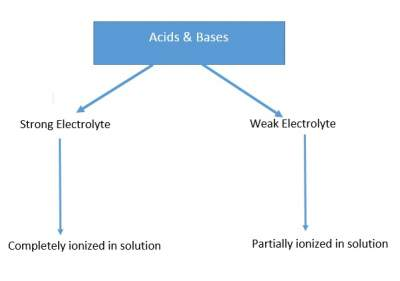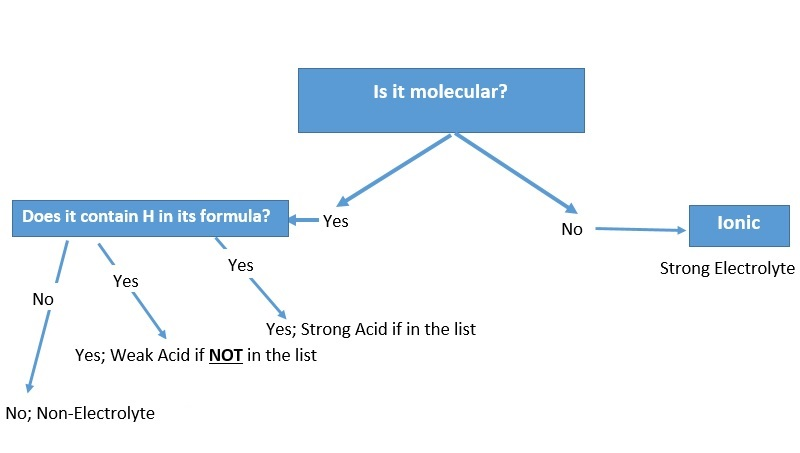# Introduction to Acid-Base Reactions

## Acid-Base Reactions

Water (H2O) is made of H+ and OH ions.
Remark:
H+ ions are H atoms that lost ONE electron leaving behind just a proton.
This explains why H+ ions are often called "protons".

### Acids

Substances that ionize in water to form Hydrogen ions (H+), so that the total [H+] concentration in water increases.

#### Examples: HCl, HNO3, H2SO4

• If acids produce ONE H+ ion if dissolved in water. These are called "Monoprotic Acids" such as HNO3
• If they ionize to TWO H+ ions, they are called "Diprotic Acids" such as H2SO4
• If they produce MORE H+ ions, they are called "Polyprotic Acid" such as H3PO4

### Bases

Substances that ionize in water to form hydroxide ions (OH), so that the total [OH] concentration in water increases.
Bases also accept (react with) H+ ions.

#### Examples: NaOH, KOH, Ca(OH)2

Remark:
Some bases do not contain (OH), but can still accept (H+) ions. They are also considered bases such as NH3

H2O + NH3 → NH4+ + OH
Acids and Bases are grouped as follows:### Strong Acids

HCl, HBr, HClO3, HI, HF is a weak acid, HNO3, H2SO4
Remark:
Because of this short list, most acids are weak.

### Strong Bases

LiOH, NaOH, KOH, RbOH, CsOH, CaOH, SrOH2, BaOH2
Remark:
These are all soluble in water. NH3 is a weak base.

To identify an electrolyte as a strong, weak or non-electrolyte? We follow the following: